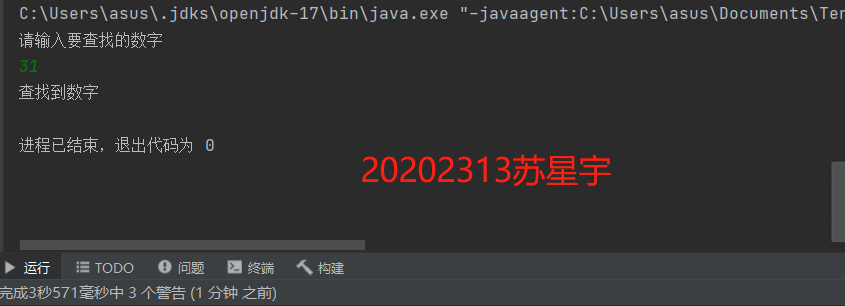1.定义一个Searching和Sorting类，并在类中实现linearSearch,SelectionSort方法，最后完成测试。要求不少于10个测试用例，提交测试用例设计情况（正常，异常，边界，正序，逆序），用例数据中要包含自己学号的后四位提交运行结果图。

2.重构你的代码把Sorting.java Searching.java放入 cn.edu.besti.cs2023.（姓名首字母+四位学号） 包中（例如：cn.edu.besti.cs1823.G2301）把测试代码放test包中重新编译，运行代码，提交编译，运行的截图（IDEA，命令行两种）

3.参考http://www.cnblogs.com/maybe2030/p/4715035.html ，学习各种查找算法并在Searching中补充查找算法并测试提交运行结果截图

4.实现排序方法等(至少3个)测试实现的算法（正常，异常，边界）提交运行结果截图（如果编写多个排序算法，即使其中三个排序程序有瑕疵，也可以酌情得满分）

5.编写Android程序对实现各种查找与排序算法进行测试提交运行结果截图推送代码到码云（选做，额外加分）

1.定义一个Searching和Sorting类，并在类中实现linearSearch,SelectionSort方法，最后完成测试。要求不少于10个测试用例，提交测试用例设计情况（正常，异常，边界，正序，逆序），用例数据中要包含自己学号的后四位提交运行结果图。public class Homework {    public static boolean linearSearch(int a,int c[]) {        for (int i = 0; i < c.length; i++) {            if (c[i] == a) {                return true;            }        }        return false;    }    public static void Selection(int a[]){        int ex=0;        for(int i=0;i<a.length;i++){            for(int j=i;j<a.length;j++){                if(a[i]>a[j]){                    ex=a[i];                    a[i]=a[j];                    a[j]=ex;                }            }        }    }}import java.util.Scanner;public class HomeworkTest {    public static void main(String[] args) {        int list[] = new int[]{23,13,95,46,85,31,54,62,1};        Scanner scan=new Scanner(System.in);        int i=0;        System.out.println("请输入要查找的数字");        i=scan.nextInt();        Homework.Selection(list);        if(Homework.linearSearch(i,list)){            System.out.println("查找到数字");        }else{            System.out.println("未查找到数字");        }    }}2.重构你的代码把Sorting.java Searching.java放入 cn.edu.besti.cs2023.（姓名首字母+四位学号） 包中（例如：cn.edu.besti.cs1823.G2301）把测试代码放test包中重新编译，运行代码，提交编译，运行的截图（IDEA，命令行两种）

测试截图

在linux中：

3.参考http://www.cnblogs.com/maybe2030/p/4715035.html ，学习各种查找算法并在Searching中补充查找算法并测试

4.实现排序方法等(至少3个)

（一）选择排序

//选择排序
public static void SelectionSort1(int a[]){
for(int i = 0; i < arr.length - 1; i++){
int minIndex = i;
for(int j = i + 1; j < a.length; j++){
if(a[j] < a[minIndex]){
minIndex = j;
}
}
swap(a, i, minIndex);
}
}
private static void swap(int a[], int i, int j) {
int temp = a[i];
a[i] = a[j];
a[j] = temp;
}

（二）冒泡排序

将待插元素，依次与已排序好的子数列元素从后到前进行比较，如果当前元素值比待插元素值大，则将移位到与其相邻的后一个位置，否则直接将待插元素插入当前元素相邻的后一位置，因为说明已经找到插入点的最终位置。

//冒泡排序
public static void MaopaoSort(int a[]) {
for (int i = 1; i < a.length; i++) {
for (int j = 0; j < a.length - 1; j++) {
if (a[j] > a[j + 1]) {
int temp = a[j];
a[j] = a[j + 1];
a[j + 1] = temp;
}
}
}
}

（三）插入排序

将待插元素，依次与已排序好的子数列元素从后到前进行比较，如果当前元素值比待插元素值大，则将移位到与其相邻的后一个位置，否则直接将待插元素插入当前元素相邻的后一位置，因为说明已经找到插入点的最终位置。

//插入排序
public static void InsertSort(int a[]) {
if (a.length >= 2) {
for (int i = 1; i < a.length; i++) {
int x = a[i];
int j = i - 1;
while (j >= 0 && a[j] > x) {
a[j + 1] = a[j];
j--;
}
a[j + 1] = x;
}
}
}

5.编写Android程序对实现各种查找与排序算法进行测试

posted on 2021-11-14 23:26  醉月居士  阅读(13)  评论(0编辑  收藏  举报